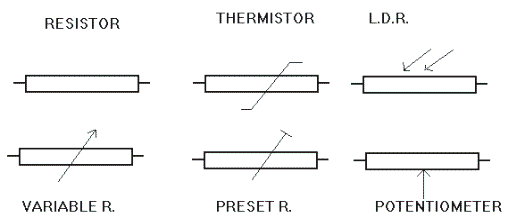Home > Electronics Tutorials > Components Tutorial > Resistor Tutorial

# Resistor Tutorial

Resistors are electronic components which resist the flow of electronic current. The higher the value of resistance (measured in ohms) the lower the current will be. This was discovered by Mr Ohm. The simplest resistors are made from carbon rod with end caps and wire leads. Other types are carbon film which is a thin layer of carbon on a ceramic rod, and metal oxide and metal glaze on glass rods. Wire wound resistors are used where the resistor has to dissipate a lot of heat.

Faulty resistors have gone open circuit or changed in value. They never go short circuit. Some resistors are designed to change in value when heated. They are called THERMISTORS and are used in temperature measuring circuits. Some resistors change in value when exposed to light. They are called LIGHT DEPENDANT RESISTORS.

Most resistors are colour coded to indicate their value and tolerance. Wire wound resistors have their value written on them. (colours would change with heat). High stability resistors (marked with a fifth pink band) do not change value easily.

Resistors generate heat. Resistors have a wattage rating. The higher this rating the more heat they can dissipate. To limit the range of resistor values to a manageable number a preferred range only is available. These are

1.0      1.2      1.8      2.2      2.7      3.3      3.9      4.7      5.6      6.8      8.2

This mean that 1 ohm, 12 ohm, 180 ohm, 2200 ohm resistors etc are available.

1000 ohms is 1k, 1000,000 ohms is 1M. 3,300,000 ohms is 3.3M etc.

Decimal points are not used on circuit diagrams (they may be confused with fly specks). 3.3M would be written as 3M3 and 1.8k as 1K8 etc.

On circuit diagrams tolerance is indicated by the following letters.

F=1% G=2% J=5% K=10% M=20%

R22M= 0.22 ohm 20% 4R7K= 4.7 ohm 10% 68RJ=68 ohm 5%Variable resistors are available. These can be operated by means of a knob on the control panel. Examples are volume and brightness controls. Preset variable resistors are internal controls which are adjusted in value by means of a screwdriver. Once adjusted, they are never touched again.